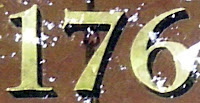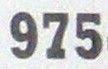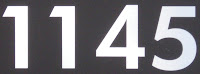## Friday, July 30, 2010

### 176

176 = 24 x 11.

176 has a unique representation as a sum of three squares: 176 = 42 + 42 + 122.

176 is the number of integer partitions of 15.

176 is the smallest number that is both a pentagonal number and an octagonal number.

Each internal angle of a regular 90-sided polygon is 176 degrees.Gliese 176 is a red dwarf star in the constellation Taurus.

## Thursday, July 29, 2010

### 241

241 is a prime number.

241 has a representation as a sum of two squares: 241 = 42 + 152.

239 and 241 form a twin prime pair.

241 is the hypotenuse of a primitive Pythagorean triple: 2412 = 1202 + 2092.

241 is 111 in base 15.

241 is the smallest prime p such that p plus the reversal of p equals a palindromic prime: 241 + 142 = 383.Reel Big Fish wrote a song entitled "241", in which the number is repeated as the only lyric.

## Wednesday, July 28, 2010

### 883

883 is a prime number.

881 and 883 form a twin prime pair.

8n + 8n + 3n is prime for n = 0 to 4: 3, 19, 137, 1051, and 8273.

883 is a number n whose fifth root has a decimal part that begins with the digits of n: 3.883221296. . .

883 is 733 in base 11 and 373 in base 16.Actress Penélope Cruz has 883 tattooed on her right ankle.

## Tuesday, July 27, 2010

### 975

975 = 3 x 52 x 13.

975 is 1111001111 in base 2 (binary).

975 is 33033 in base 4, 4303 in base 6, 1303 in base 9, and 303 in base 18.

975 is a number that cannot be written as a sum of three squares.

975 is the number of 11-ominoes that contain one hole.The Wright R-975 Whirlwind was a radial engine developed in the United States by Wright Aeronautical.

## Monday, July 26, 2010

### 778

778 = 2 x 389.

778 has a representation as a sum of two squares: 778 = 72 + 272.

778 is the number of ways a 5 x 1 rectangle can be surrounded by 5 x 1 rectangles.

778 is a Smith number. The sum of its digits is equal to the sum of the digits of its prime factors: 7 + 7 + 8 = 2 + 3 + 8 + 9.778 is a telephone area code for British Columbia.

## Friday, July 23, 2010

### 677

677 is a prime number.

677 has a representation as a sum of two squares: 677 = 12 + 262.

677 is the sum of three consecutive squares: 677 = 142 + 152 + 162.

677 is the hypotenuse of a primitive Pythagorean triple: 6772 = 522 + 6752.677 Aaltje is an asteroid named for a Dutch singer.

## Thursday, July 22, 2010

### 88

88 = 23 x 11.

88 has a unique representation as a sum of three squares: 88 = 42 + 62 + 62.

88 = 3 + 4 + 5 + 6 + 7 + 8 + 9 + 10 + 11 + 12 + 13.

88 is the sixth number that stays the same when written upside down.

88 is the smallest number such that its square has all its digits twice: 882 = 7744.A modern piano has 88 keys, 52 white and 36 black, spanning more than seven octaves.

88 is the number of constellations in the sky, as defined by the International Astronomical Union.

Source: Phillips, R. 1994. Numbers: Facts, Figures and Fiction. Cambridge University Press.

## Wednesday, July 21, 2010

### 773

773 is a prime number.

773 has a representation as a sum of two squares: 773 = 172 + 222.

773 is the hypotenuse of a primitive Pythagorean triple: 7732 = 1952 + 7482.

773 is the largest three-digit unholey prime; its digits have no holes.

773 is the smallest prime such that adding a preceding or trailing digit will always result in a composite if we omit the case where the leading digit is zero.773 is a telephone area code for Chicago.

## Tuesday, July 20, 2010

### 1145

1145 = 5 x 229.

1145 has two representations as a sum of two squares: 1145 = 112 + 322 = 192 + 282.

1145 is the hypotenuse of two Pythagorean triples: 11452 = 4232 + 10642 = 7042 + 9032.

1145, 1147 (31 x 37), and 1149 (3 x 383) are each semiprimes, the product of two primes.Halley's Comet appeared in the skies in the year 1145.

Source: WolframAlpha

## Monday, July 19, 2010

### 137

137 is a prime number.

137 has a representation as a sum of two squares: 137 = 42 + 112.

137 and 139 form a twin prime pair.

137 is the hypotenuse of a primitive Pythagorean triple: 1372 = 882 + 1052.

137 is a divisor of 374 - 1.

137 = 128 + 8 + 1 = 27 + 23 + 20.A molecule of chlorophyll a (C55H72O5N4Mg) consists of 137 atoms.

## Friday, July 16, 2010

### 272

272 = 24 x 17.

272 has a representation as a sum of two squares: 272 = 42 + 162.

272 is the number of hyperspheres in 9 dimensions that can touch an equivalent hypersphere without any intersections (Kissing Number).

272 is a pronic number, the product of two consecutive numbers: 272 = 16 x 17.

All the digits of 272 are prime, and the sum of the digits of this palindromic composite is 11, a palindromic prime. The product of the digits is 28, a perfect number.

272 is the sum of four consecutive primes: 272 = 61 + 67 + 71 + 73.A Calorie Counter offers a list of the 272 fast food items highest in calories.

## Thursday, July 15, 2010

### 441

441 = 32 x 72.

441 = 212, a square.

441 is the smallest square that is the sum of six consecutive cubes: 441 = 13 + 23 + 33 + 43 + 53 + 63.

441 = 212 and 144 = 122. Also, 441 x 144 = 2522.441 is the telephone area code for the island of Bermuda.

## Wednesday, July 14, 2010

### 344

344 = 23 x 43.

344 is the maximum number of regions into which 19 circles divide the plane.

The sum of the squares and the sum of the cubes of the factors of 344 are both primes: 22 + 22 + 22 + 432 = 1861 and 23 + 23 + 23 + 433 = 79531.In the year 344 B.C., the Greek philosopher Aristotle traveled from the town of Assos to the island of Lesbos to study natural history.

## Tuesday, July 13, 2010

### 528

528 = 24 x 3 x 11.

528 is 1000010000 in base 2 (binary).

528 is the 32nd triangular number.

528 concatenated with its successor is a square: 528529 = 7272.

528 is 646 in base 9.Florida State Road 528 (SR 528) connects Orlando with Cape Canaveral.

## Monday, July 12, 2010

### 243

243 = 35, a perfect fifth power.

243 is the first number of the form pq where p and q are a twin prime pair (3, 5).

243 is the sum of the first three palindromic primes: 243 = 11 + 101 + 131.

243 is 3303 in base 4, 1433 in base 5, 1043 in base 6, 363 in base 8, 300 in base 9, 183 in base 12, and 113 in base 15.243 Ida is a large, irregularly shaped asteroid. It was the second asteroid to be visited by a spacecraft and the first to be found to possess a satellite.